# Windows 8: Use Calculator App to Perform Date Calculations

Are you counting down the days until that concert you have been dying to see? Do you want to know how many days you have been alive? Whatever your motives are, the Windows 8 calculator application comes with a built-in date calculator.

This tech-recipe will cover both day countdowns and adding/subtracting from a specific date.

1.To start the calculations, we will have to first open up the Calculator applications. Search for it from the Start screen.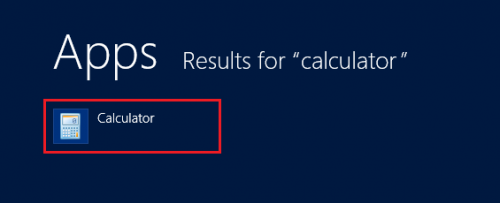2.From the Calculator application, select View, and then choose Date Calculation. To go directly to it, you can also press Ctrl + E from the first Calculator screen.3.First, I will show you how you can calculate the number of days between two dates. Select both From and To dates. For example, if you are trying to calculate the number of days you have been alive, you would select your birthday and today’s date.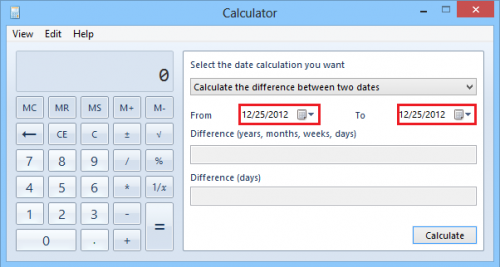4.When you have selected both dates, you can now click Calculate.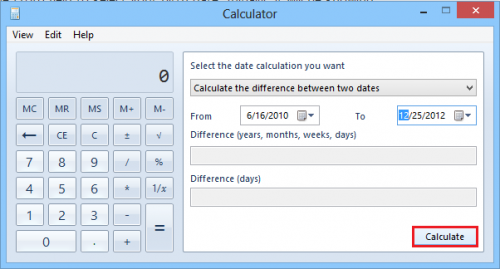As you can see, you are given an answer in both a years, months, weeks and days format along with just a traditional day count.5.This part of the tutorial will cover adding or subtracting from a certain date. To do so, we will need to select Add or subtract days to a specified date from the list of calculations.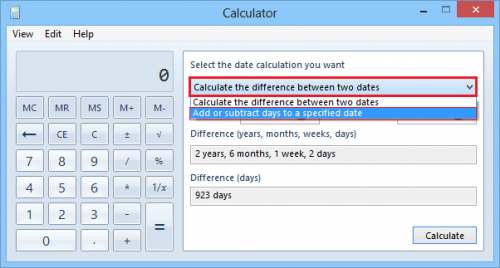6.Now, select the date from which you want to add or subtract. Then choose Add or Subtract, and enter the amount of years / months / days. Then hit Calculate.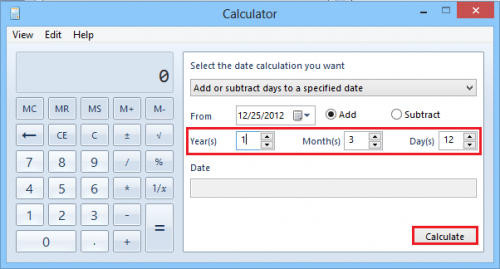You are presented with the calculated date.